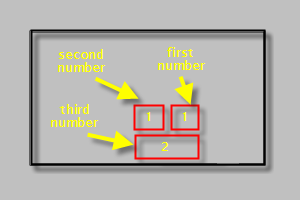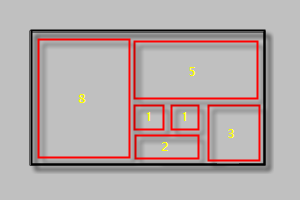Fine Art Photography in the Digital Era

# EYE6: Geometric Series and Framing

In the last article we discussed the Golden Rule and it’s application in dividing a Frame. We found that such a division gave us both a point to place smaller objects on a large background and a four panel division to place larger objects. The whole point of the Golden Rule was an aide in placing the subject.

## Definitions

Another such division aide is the Geometric Series. A Geometric Series is a list of numbers in which each number in the series has the same ratio from the previous number to the next number. For example, start with the number 1 and add 3 each time. Our series is 1, 4, 7, 10, 13 … If you were to graph this series of numbers, you would end up with a curve, not a straight line. Hence, this is where the name Geometric Series comes from.

The most common form of a Geometric Series is the Fibonacci Series. Instead of picking a constant number, the series adds the previous two numbers together and that result becomes the next number in the series. I.e., 1, (1 + 0 = 1) 1, (1 + 1 = 2) 2, (1 + 2 = 3) 3, (2 + 3 = 5) 5, (3 + 5 = 8) 8 … The progression is much faster than the previous example. Also notice that the first two numbers are the same: 1. This is because of the value zero.

## Using to Divide a Frame

Now that we see a formula that is used for calculating a Geometric Series, how do we use that to divide a frame? Obviously, this is arbitrary, so we need to define a measurement to apply to the numbers in the series. Let’s start simple and get more complicated. Take 1 unit as 1/10th of the size of the frame, square. Thus 1 = 1/10th x 1/10th of the frame.Then, after having a unit established, we add the first couple of numbers in the series: 1, 1, 2.Finally, after filling the frame through the sixth number in the series, we get a division like the following:If you look at the final division of the Frame, you can begin to see the seashell spiral that is commonly associated with the Fibonacci Series. This is also known as the Golden Spiral.

## Application

Certainly, one would not have the time to create such a division and frame a photograph when working in photojournalism and street photography. But the speed which one shoots an image does not determine whether this type of Frame division is applicable. The more global point is the concept of a smaller portion of the subject offset near the same point as the Golden Rule creates. This would be in the area of the two number 1 boxes or divisions. Then as the subject and background blend together, try to create a uniform feeling of moving down, out and around. Leave the larger portions of the subject or the background to fill the second half of the Frame, or block 8.

Practicing with still life gives you the opportunity to train your eye to use this complex, mathematical formula in your frame. This is the most challenging portion our series on Visualization and if you don’t quite understand the math, that is ok. The feeling of the division, the flowing movement that the Geometric Series gives when applied to the Frame is what we are after, not the calculation of a Fibonacci Series.

## Exercises

As we did in the last article, take a clear overhead sheet or a page protector and draw out the proportions of your camera Frame. Divide the Frame into a Fibonacci series by starting in the reverse, with number 8 as half the image. Then move backwards through to the two number 1 blocks.

Drawing this on a clear sheet will not only give you an overlay to view with your Eyes, but also an easy method of flipping the division and using the mirrored image with the number 8 block on the right instead of the left.

Using this tool, find four subjects and take at least three photographs of each one. In the photographs try to implement the Divisions you created. Use people, buildings, clouds, trees, objects of any kind to fill in your Frame divisions.

Print the images and make notes of how successful you were. Take a ruler and a colored pen or pencil and draw the Fibonacci Series directly on the photograph. When finished, place these in your scrapbook with the others.

Repeating this exercise over and over with the Golden Ratio exercise and the Linear Divisions exercise will train your eye to begin seeing in this manner.